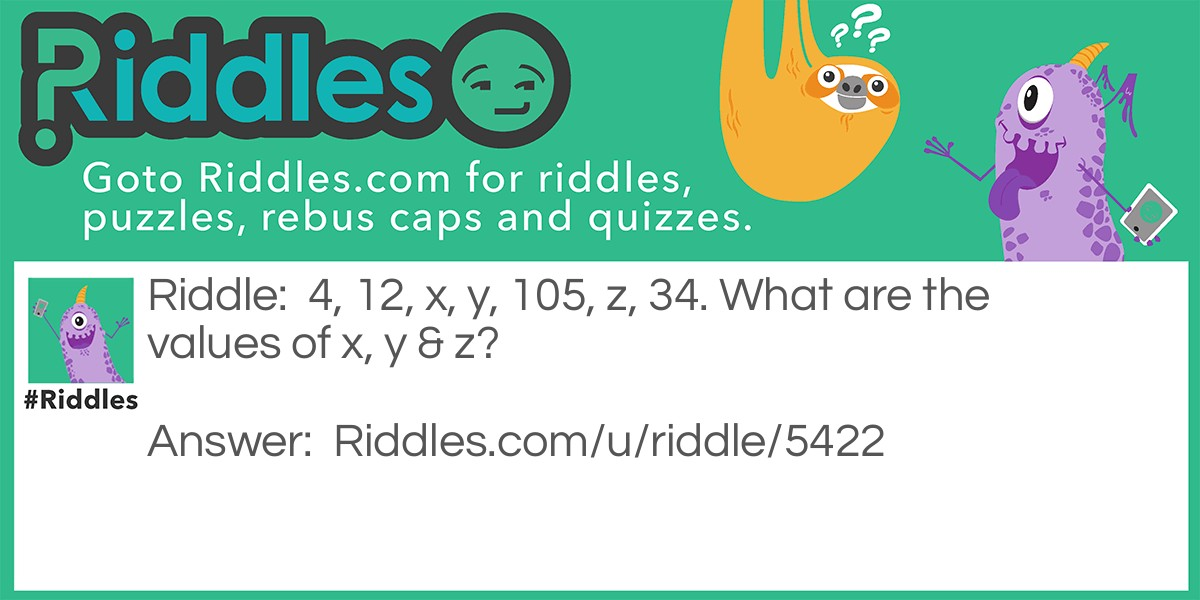ADVERTISEMENT
Riddle:

4, 12, x, y, 105, z, 34. What are the values of x, y & z?

Answer:

x=36, y=108, z=102, To get x & y, multiply the preceding number by 3 to get the next value. But after y, the next numbers decrease by 3, So z=105-3=102, then at z, the numbers are divided by 3.

VOTE
Rated 3/5 based on 57 votes
SHARE
COMMENTMaths Riddles 4 | Short Riddle Riddle Meme with riddle and answer page link.
ADVERTISEMENT